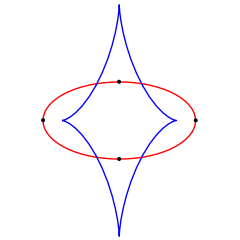# Vertex (curve)

Not to be confused with Vertex (geometry).
For other uses, see Vertex (disambiguation).An ellipse (red) and its evolute (blue). The dots are the vertices of the curve, each corresponding to a cusp on the evolute.

In the geometry of planar curves, a vertex is a point of where the first derivative of curvature is zero. This is typically a local maximum or minimum of curvature, and some authors define a vertex to be more specifically a local extreme point of curvature. However, other special cases may occur, for instance when the second derivative is also zero, or when the curvature is constant. For space curves, on the other hand, a vertex is a point where the torsion vanishes.

## Examples

A hyperbola has two vertices, one on each branch; they are the closest of any two points lying on opposite branches of the hyperbola, and they lie on the principal axis. On a parabola, the sole vertex lies on the axis of symmetry. On an ellipse, two of the four vertices lie on the major axis and two lie on the minor axis.

For a circle, which has constant curvature, every point is a vertex.

## Cusps and osculation

Vertices are points where the curve has 4-point contact with the osculating circle at that point. In contrast, generic points on a curve typically only have 3-point contact with their osculating circle. The evolute of a curve will generically have a cusp when the curve has a vertex; other, more degenerate and non-stable singularities may occur at higher-order vertices, at which the osculating circle has contact of higher order than four. Although a single generic curve will not have any higher-order vertices, they will generically occur within a one-parameter family of curves, at the curve in the family for which two ordinary vertices coalesce to form a higher vertex and then annihilate.

The symmetry set of a curve has endpoints at the cusps corresponding to the vertices, and the medial axis, a subset of the symmetry set, also has its endpoints in the cusps.

## Other properties

According to the classical four-vertex theorem, every simple closed planar smooth curve must have at least four vertices. A more general fact is that every simple closed space curve which lies on the boundary of a convex body, or even bounds a locally convex disk, must have four vertices.

If a planar curve is bilaterally symmetric, it will have a vertex at the point or points where the axis of symmetry crosses the curve. Thus, the notion of a vertex for a curve is closely related to that of an optical vertex, the point where an optical axis crosses a lens surface.

1. Agoston (2005), p. 570; Gibson (2001), p. 126.
2. Gibson (2001), p. 127.
3. Fuks & Tabachnikov (2007), p. 141.
4. Agoston (2005), p. 570; Gibson (2001), p. 127.
5. Gibson (2001), p. 126.
6. Fuks & Tabachnikov (2007), p. 142.
7. Agoston (2005), Theorem 9.3.9, p. 570; Gibson (2001), Section 9.3, "The Four Vertex Theorem", pp. 133–136; Fuks & Tabachnikov (2007), Theorem 10.3, p. 149.
• Agoston, Max K. (2005), Computer Graphics and Geometric Modelling: Mathematics, Springer, ISBN 9781852338176.
• Fuks, D. B.; Tabachnikov, Serge (2007), Mathematical Omnibus: Thirty Lectures on Classic Mathematics, American Mathematical Society, ISBN 9780821843161
• Ghomi, Mohammad (2015), Boundary torsion and convex caps of locally convex surfaces, arXiv:1501.07626• Gibson, C. G. (2001), Elementary Geometry of Differentiable Curves: An Undergraduate Introduction, Cambridge University Press, ISBN 9780521011075.
• Sedykh, V.D. (1994), "Four vertices of a convex space curve", Bull. London Math. Soc., 26 (2): 177–180